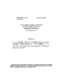## Two Simple Residual Bounds for the Eigenvalues of Hermitian Matrices##### Files
CS-TR-2364.ps(90.65 KB)
Let $A$ be Hermitian and let the orthonormal columns of $X$ span an approximate invariant subspace of $X$. Then the residual $R = AX-XM$ $(M=X\ctp AX)$ will be small. The theorems of this paper bound the distance of the spectrum of $M$ from the spectrum of $A$ in terms of appropriate norms of $R$. Appeared in SIAM J. Mat. Anal. Appl. 12 (1991) 205--208. (Also cross-referenced as UMIACS-TR-89-123)